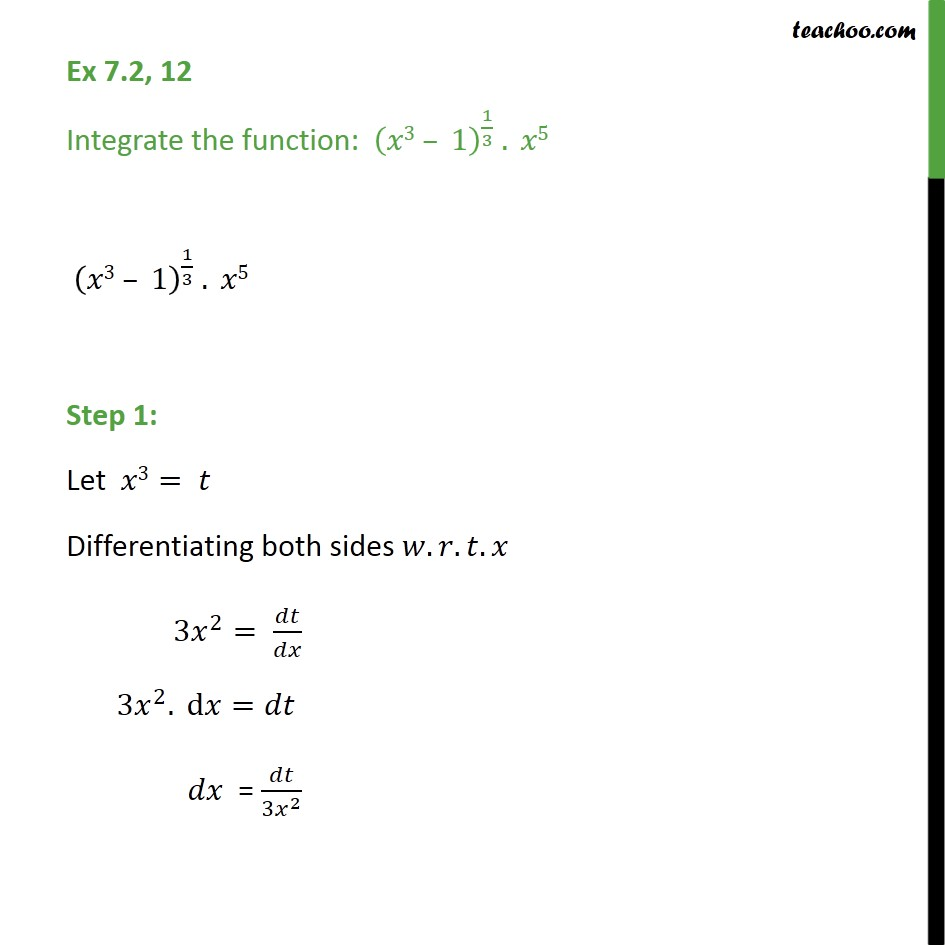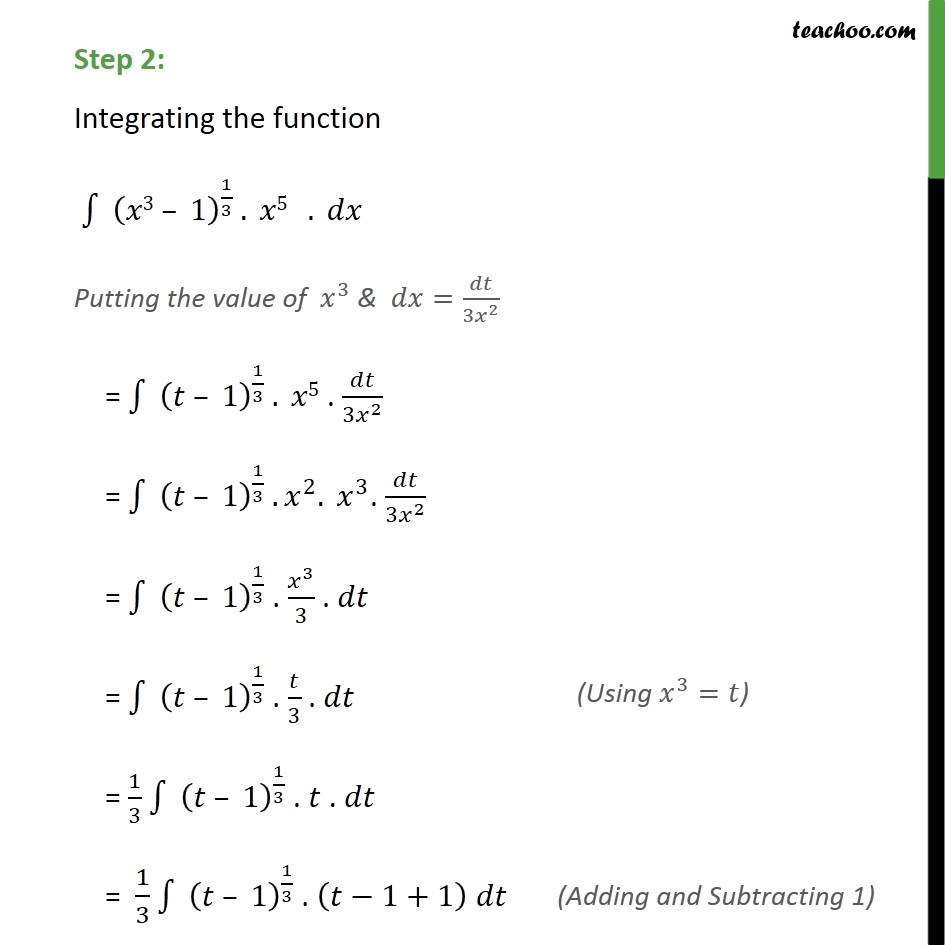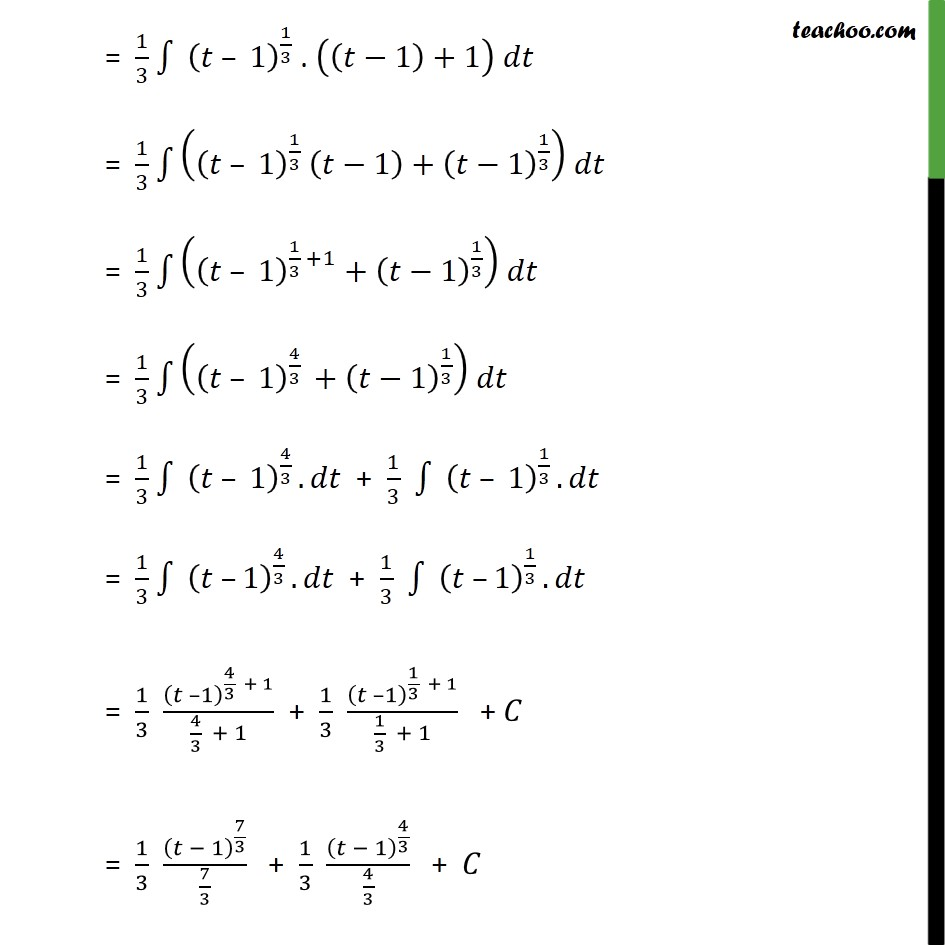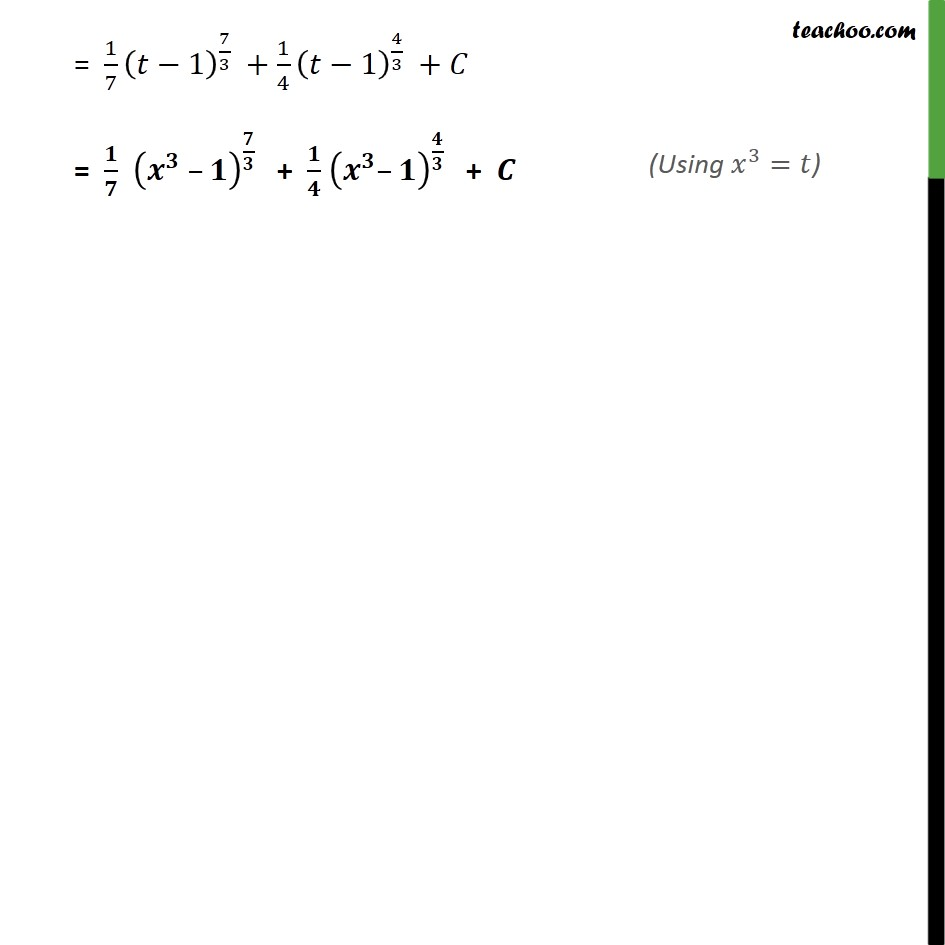Ex 7.2

Chapter 7 Class 12 Integrals
Serial order wiseLearn in your speed, with individual attention - Teachoo Maths 1-on-1 Class

### Transcript

Ex 7.2, 12 Integrate the function: (𝑥3 – 1)^(1/3) . 𝑥5 (𝑥3 – 1)^(1/3) . 𝑥5 Step 1: Let 𝑥3= 𝑡 Differentiating both sides 𝑤.𝑟.𝑡.𝑥 3𝑥^2= 𝑑𝑡/𝑑𝑥 3𝑥^2. d𝑥=𝑑𝑡 𝑑𝑥 = 𝑑𝑡/(3𝑥^2 ) Step 2: Integrating the function ∫1▒〖" " (𝑥3 – 1)^(1/3) . 𝑥5" " 〗 . 𝑑𝑥 Putting the value of 𝑥^3 & 𝑑𝑥=𝑑𝑡/(3𝑥^2 ) = ∫1▒〖" " (𝑡 – 1)^(1/3) . 𝑥5〗 . 𝑑𝑡/(3𝑥^2 ) = ∫1▒〖" " (𝑡 – 1)^(1/3) . 𝑥^2. 𝑥^3 〗. 𝑑𝑡/(3𝑥^2 ) = ∫1▒〖" " (𝑡 – 1)^(1/3) 〗 . 𝑥^3/3 . 𝑑𝑡 = ∫1▒〖" " (𝑡 – 1)^(1/3) 〗 . 𝑡/3 . 𝑑𝑡 = 1/3 ∫1▒〖" " (𝑡 – 1)^(1/3) 〗 . 𝑡 . 𝑑𝑡 = 1/3 ∫1▒〖" " (𝑡 – 1)^(1/3) 〗 . (𝑡−1+1) 𝑑𝑡 = 1/3 ∫1▒〖" " (𝑡 – 1)^(1/3) 〗 . ((𝑡−1)+1) 𝑑𝑡 = 1/3 ∫1▒((𝑡 – 1)^(1/3) (𝑡−1)+(𝑡−1)^(1/3) ) 𝑑𝑡 = 1/3 ∫1▒((𝑡 – 1)^(1/3 +1)+(𝑡−1)^(1/3) ) 𝑑𝑡 = 1/3 ∫1▒((𝑡 – 1)^(4/3 )+(𝑡−1)^(1/3) ) 𝑑𝑡 = 1/3 ∫1▒〖 (𝑡 – 1)^(4/3 ). 𝑑𝑡〗 + 1/3 ∫1▒〖 (𝑡 – 1)^(1/3 ). 𝑑𝑡〗 = 1/3 ∫1▒〖 (𝑡 –1)^(4/3 ). 𝑑𝑡〗 + 1/3 ∫1▒〖 (𝑡 –1)^(1/3 ). 𝑑𝑡〗 = 1/3 (𝑡 –1)^(4/3 + 1)/(4/3 + 1) + 1/3 (𝑡 –1)^(1/3 + 1)/(1/3 + 1) + 𝐶 = 1/3 (𝑡 − 1)^(7/3)/(7/3) + 1/3 (𝑡 − 1)^(4/3)/(4/3) + 𝐶 = 1/7 (𝑡−1)^(7/3) +1/4 (𝑡−1)^(4/3) +𝐶 = 𝟏/𝟕 (𝒙^𝟑 –𝟏)^(𝟕/𝟑) + 𝟏/𝟒 (𝒙^𝟑–𝟏)^(𝟒/𝟑) + 𝑪